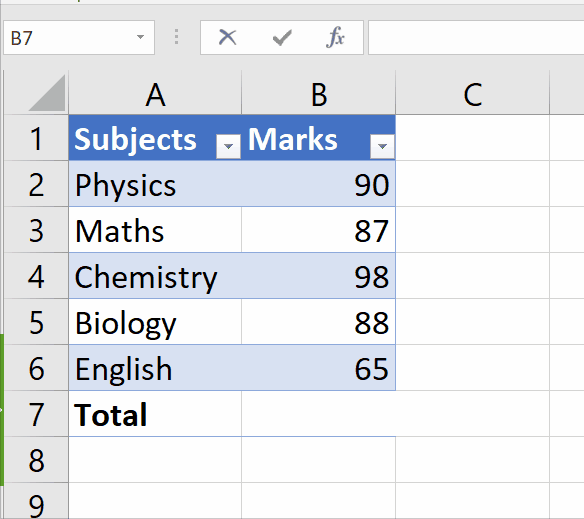# How to add cells in Excel

In this tutorial, we’ll learn how to add cells in Excel. We can add values, individual values, cell references, ranges, and mix of all. There is a special formula of sum, which is;

=sum(value1 : value2)

Value 1 means the first value of range and value 2 is the last value of range.

To sum up by writing the formula in Excel, the steps are pretty much easier and most commonly used. However, to achieve this goal please follow the steps below;Excel offers incredible features to ease mathematical calculations by using built-in formulas. One of those features is addition/sum, which enables us to calculate the sum of many numbers and simplify the calculation process with just one click.

### Step 1 – Select the data range– Go to the blank cell, where you wish to make the sum of your desired range.
– Type the formula, in our case the selected cells are B2 and B6 hence, formula would be =SUM(B2:B6).
– As soon as you press the enter key, it’d add all the cells of the data range.

To do at yourself, above is the animation as an example;

To conclude, we can see in animation, cells of data range have been added, formula is shown in the formula bar, and the result is displayed.